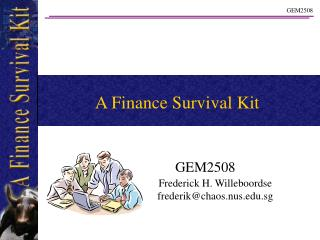DownloadDownload PresentationA Finance Survival Kit

# A Finance Survival Kit

Télécharger la présentation## A Finance Survival Kit

- - - - - - - - - - - - - - - - - - - - - - - - - - - E N D - - - - - - - - - - - - - - - - - - - - - - - - - - -
##### Presentation Transcript

1. A Finance Survival Kit GEM2508 Frederick H. Willeboordse frederik@chaos.nus.edu.sg

2. Lecture 6 Break Even Analysis

3. Fixed versus Variable Costs Break Even Analysis Today’s Lecture

4. Break-Even Analysis An important part of running a company is the determination of how the company should be financed and how the prices of the products the company sells should be set. Clearly, in order to do this properly a sound financial analysis is necessary.

5. Types of Costs • It is essential to realize that there are two basic types of costs a company incurs. • Variable Costs • Fixed Costs • Variable costs are roughly proportional to a company’s sales. I.e. the cost per unit remains roughly constant. • Fixed costs remain roughly the same regardless of sales. I.e. the cost per unit is inversely proportional to sales.

6. Types of Costs Sales Value Total Costs Variable Costs Fixed Costs Sales A simple graph but important to understand!

7. Break-Even Analysis Commonly, the Break-even point is defined to be the level of sales where: Revenues = Expenses Let us have a look at a simple example. Aunt Petunia opens a flower shop.

8. Break-Even Analysis • Fixed Costs: • Rent: 5,000 • Utilities: 300 • Helper: 1,500 • Variable Costs: • Flowers: 40% of selling price • So we know that: • Selling price – cost of flowers – rent – utilities – helper = 0 • when she breaks even

9. Break-Even Analysis Let’s enter the last line into the spread sheet =E6-E7-E8-E9-E10 =E6*\$H\$7 But!!!?? I’m not a Mathematician! How am I going to find the value for E6 so that E11 = 0? It’s good to put the assumptions separately.

10. Break-Even Analysis Piece of cake! Trial and error! Oops! Not as easy as I thought. Especially if H7 is not a nice number like 40%. Is there a better way?

11. The Solver We could cave and write down the equation. That would, in fact not be such a bad idea since equations are really not as difficult to understand when one knows what they are supposed to mean. But *** RELIEF *** we can use the previously discussed solver. That was close ….

12. Break-Even Analysis Lets use the Solver! The break even point is 11,333 Incredible! Like this anyone can do Math!

13. An Equation … • The Solver is a fabulous tool, but really in business it is beneficial to at least be open-minded about equations. • Let’s give it a try! We know that: • E11=E6-E7-E8-E9-E10 • (Income = Sales – Cost – Rent – Utilities – Helper) • At the break even point, Net Income = 0 and hence E11 = 0 • Inserting this we obtain: • 0 = E6-E7-E8-E9-E10

14. An Equation … • We want to change E6 such that • 0 = E6-E7-E8-E9-E10 • is true. • Ah, if we just set E6 to the other values together the above equation is true. Or, • E6 = E7+E8+E9+E10 • is the solution to our problem. • Let’s try it and go back to the situation before we used the solver:

15. Break-Even Analysis Oops! Let’s fill the equation into cell E6 =E7+E8+E9+E10 What went wrong?

16. An Equation … May be Math isn’t as easy as I thought… But wait, doesn’t E7 depend on E6? Yes indeed, we have defined E7 = E6 * H7 (cost of product). Let’s put this into our equation: E6 = E7+E8+E9+E10 => E6 = E6*H7+E8+E9+E10 Or: E6 - E6*H7 = E8+E9+E10 E6 * (1 - H7) = E8+E9+E10 E6 = (E8+E9+E10) / (1 - H7)

17. Break-Even Analysis Yatta! It works Yosh! Let’s try that again. (E8+E9+E10) / (1 - H7) After all, this was quite easy wasn’t it? And now we can change H7 to play out scenarios. Very useful indeed!

18. Break-Even Analysis If Aunt Petunia raises the price so that the cost is only 30% of sales …. … she can break even already at 9714. Of course now she may be so expensive that no one wants to buy her flowers anymore.

19. The Other Way Around There may be times when it is betterto turn things around. Just now, Aunt Petunia found how much she needs to sell in order to break even givencertain cost as percentage of sales. This is in general a good idea since for many products the profits are more or less set. In order to see how feasible a business is one could also enter an expected sales amount and then see how much the cost of the product can be in order to break even.

20. Break-Even Analysis • With our nice equation, it’s fairly easy to accomplish this: • E6 * (1 - H7) = E8+E9+E10 • (1 – H7) = (E8+E9+E10)/E6 • H7 = 1 - (E8+E9+E10)/E6 Cool! And I thought that Financial Analysis is only for … others 

21. The Solver is a great tool But Math can be very useful as well There are two types of costs: Variable Costs Fixed Costs Break Even Analysis is a snap with Excel Key Points of the Day